﻿ 基于误差补偿的高精度微光双目测距

基于误差补偿的高精度微光双目测距High Precision Low-Light-Level Binocular Range Measurement Based on Error Compensation

Abstract: In order to realize long-distance and high-precision binocular ranging measurement in low-light-level environment, based on binocular parallax principle and triangulation reconstruction method, in the light of characteristics of low-light-level imaging and general analysis of binocular ranging methods, this thesis constructs a portable low-light-level ranging prototype system using stable and efficacious feature extraction method adaptive for low-light-level image, and implements a system accuracy optimization method. Experiments show that the method is highly robust and precise. In 20~200 meters, the relative error of the corrected results is below 1%, and response time less than 0.05 s.

1. 引言

2. 系统开发环境与设计流程图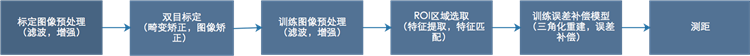Figure 1. System algorithm flow chart

3. 双目测距系统的标定

$\left\{\begin{array}{l}u=\lambda X\\ v=\lambda Y\\ {f}^{\prime }=\lambda Z\end{array}⇒\left\{\begin{array}{l}u={f}^{\prime }\frac{X}{Z}\\ v={f}^{\prime }\frac{Y}{Z}\end{array}$ (1)

4. 基于三角化的双目测距方法

4.1. 微光图像匹配

GMS特征匹配将高匹配数字转换成高匹配质量，提供了实时、高精度的匹配系统，符合本文对测距实时性的要求。在评估低质量、模糊的图像上，GMS效果优于其他实时匹配器。经过预处理后的图像可以得到较为精确的同名点坐标，解决了通常微光图像无法进行特征匹配的难题。

4.2. 三维重建和三角化测量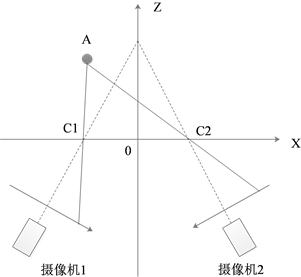Figure 2. Converged binocular stereoscopic imaging relationship diagram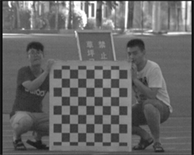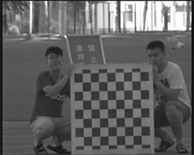(a)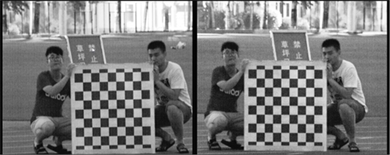(b)

Figure 3. Collected (a) low-light-level image and (b) the preprocessed imageFigure 4. GMS feature matching result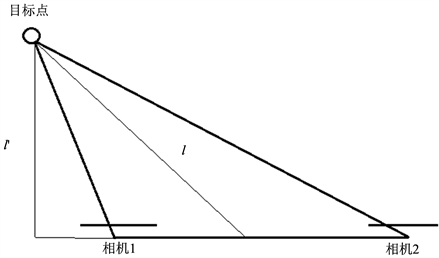Figure 5. Measurement of distance difference between two binocular range methods. When the range is far away, the target in the image public area has a long distance to move. And there is negligible difference in the representation of the two methods

$p=HP$ (2)

$p=s\left[u,v,1\right]$ 为在相机成像平面上的广义坐标， $s$ 为未知的尺度参数，现在定义 ${H}_{1},{H}_{2},{H}_{3}$$H$ 矩阵的行向量，等式(2)可以重新写为：

$su={H}_{1}^{\text{T}}P,\text{\hspace{0.17em}}\text{\hspace{0.17em}}sv={H}_{2}^{\text{T}}P,\text{\hspace{0.17em}}\text{\hspace{0.17em}}s={H}_{3}^{\text{T}}P$ (3)

$u{H}_{3}^{\text{T}}P={H}_{1}^{\text{T}}P,\text{\hspace{0.17em}}\text{\hspace{0.17em}}u{H}_{3}^{\text{T}}P={H}_{2}^{\text{T}}P$ (4)

$\left[\begin{array}{c}{u}_{l}{\left({K}_{l}R\right)}_{3}^{\text{T}}-{\left({K}_{l}R\right)}_{1}^{\text{T}}\\ {v}_{l}{\left({K}_{l}R\right)}_{3}^{\text{T}}-{\left({K}_{l}R\right)}_{2}^{\text{T}}\\ {u}_{r}{\left({K}_{r}R\right)}_{3}^{\text{T}}-{\left({K}_{r}R\right)}_{1}^{\text{T}}\\ {v}_{r}{\left({K}_{r}R\right)}_{3}^{\text{T}}-{\left({K}_{r}R\right)}_{2}^{\text{T}}\end{array}\right]P=\left[\begin{array}{c}{u}_{l}{\left({K}_{l}t\right)}_{3}^{\text{T}}-{\left({K}_{l}t\right)}_{1}^{\text{T}}\\ {u}_{l}{\left({K}_{l}t\right)}_{3}^{\text{T}}-{\left({K}_{l}t\right)}_{2}^{\text{T}}\\ {u}_{r}{\left({K}_{r}t\right)}_{3}^{\text{T}}-{\left({K}_{r}t\right)}_{1}^{\text{T}}\\ {u}_{r}{\left({K}_{r}t\right)}_{3}^{\text{T}}-{\left({K}_{r}t\right)}_{2}^{\text{T}}\end{array}\right]$ (5)

$\left({u}_{l},{v}_{l}\right),\left({u}_{r},{v}_{r}\right)$ 为左右目相机成像平面的匹配点坐标， ${K}_{l},{K}_{r}$ 为相机内参数矩阵，因为坐标系是以左目成像平面为原点的，所以等式中相对于左目的 $R$ 为单位矩阵， $t$ 为0向量，等式简化后可得：

$\left[\begin{array}{c}{u}_{l}{K}_{l3}^{\text{T}}-{K}_{l1}^{\text{T}}\\ {v}_{l}{K}_{l3}^{\text{T}}-{K}_{l2}^{\text{T}}\\ {u}_{r}{\left({K}_{r}R\right)}_{3}^{\text{T}}-{\left({K}_{r}R\right)}_{1}^{\text{T}}\\ {v}_{r}{\left({K}_{r}R\right)}_{3}^{T}-{\left({K}_{r}R\right)}_{2}^{\text{T}}\end{array}\right]P=-\left[\begin{array}{c}0\\ 0\\ {u}_{r}{\left({K}_{r}t\right)}_{3}^{\text{T}}-{\left({K}_{r}t\right)}_{1}^{\text{T}}\\ {u}_{r}{\left({K}_{r}t\right)}_{3}^{\text{T}}-{\left({K}_{r}t\right)}_{2}^{\text{T}}\end{array}\right]$ (6)

5. 建立误差补偿模型

$f\left(x\right)=a+b\ast x+c\ast {x}^{2}+d\ast {x}^{3}$ (7)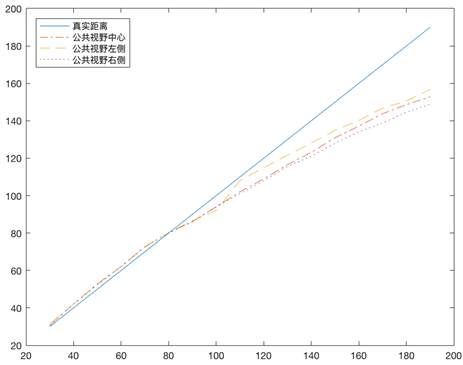Figure 6. The error relation diagram between the measured value and the true value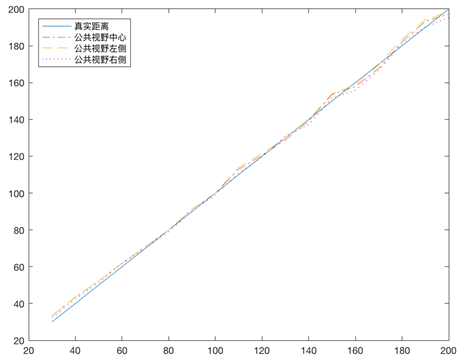Figure 7. The result map of distance measurement after error compensation

6. 测距系统实验结果

${K}_{1}=\left[\begin{array}{ccc}1.1203080461376\text{e}+004& 0& 3.5950000000000\text{e}+002\\ 0& 1.1325344549237\text{e}+004& 2.8750000000000\text{e}+002\\ 0& 0& 1\end{array}\right]$ (8)

${K}_{2}=\left[\begin{array}{ccc}1.0547975764754\text{e}+004& 0& 3.5950000000000\text{e}+002\\ 0& 1.0779346493816\text{e}+004& 2.8750000000000\text{e}+002\\ 0& 0& 1\end{array}\right]$ (9)

$R=\left[\begin{array}{ccc}0.9996888402636573& -0.02412153879231011& 0.006354055287352623\\ 0.02412854691842225& 0.9997083350816923& -0.001028587281730117\\ -0.006327390924318782& 0.001181581347905892& 0.9999792837802237\end{array}\right]$ (10)

$t={\left[\begin{array}{ccc}-1540.583996830434& -42.60545288611834& -1354.669755047537\end{array}\right]}^{\text{T}}$ (11)

${K}_{1}$${K}_{2}$ 位左右目相机的内参数， $R$ 是右目相对于左目相机的旋转矩阵， $t$ 是右目相对于左目相机的平移矩阵。拟合多项式如式(12)：

$f\left(x\right)=7.2666+0.3012x+0.007{x}^{2}-0.000004{x}^{3}$ (12)Table 2. Comparison of the results of four kinds of distance measurement

7. 结语

 杨照金. 军用目标伪装隐身技术概论[M]. 北京: 国防工业出版社, 2014.

 胡海波, 邱民仆. 微光图像对比度增强技术研究[J]. 测绘通报, 2015(1): 26-30.

 胡迈, 曹维国, 王劲松, 安志勇, 林婉宜, 于丽婷. 微光夜视环境中自然微光环境模拟[J]. 长春理工大学学报(自然科学版), 2015, 38(1): 29-33.

 裴思惠. 高精度双目测距系统标定方法研究[D]: [硕士学位论文]. 长春: 长春理工大学, 2016.

 姜雨彤. 双目测距系统及标定方法研究[D]: [硕士学位论文]. 长春: 长春理工大学, 2013.

 马继红, 陈安方, 赵玉芹. 基于立体视觉的被动测距技术研究[J]. 西南师范大学学报(自然科学版), 2016, 41(9): 94-99.

 马源. 基于双目立体视觉的深度感知技术研究[D]: [硕士学位论文]. 北京: 北京理工大学, 2015.

 李明. 低照度图像增强技术的研究及实现[D]: [硕士学位论文]. 南京: 南京邮电大学, 2016.

 Bian, J.W., Lin, W.Y., Matsushita, Y., et al. (2017) GMS: Grid-Based Motion Statistics for Fast, Ultra-Robust Feature Correspondence. IEEE Conference on Computer Vision and Pattern Recognition, Honolulu, 21-26 July 2017, 2828-2837.
https://doi.org/10.1109/CVPR.2017.302

Top